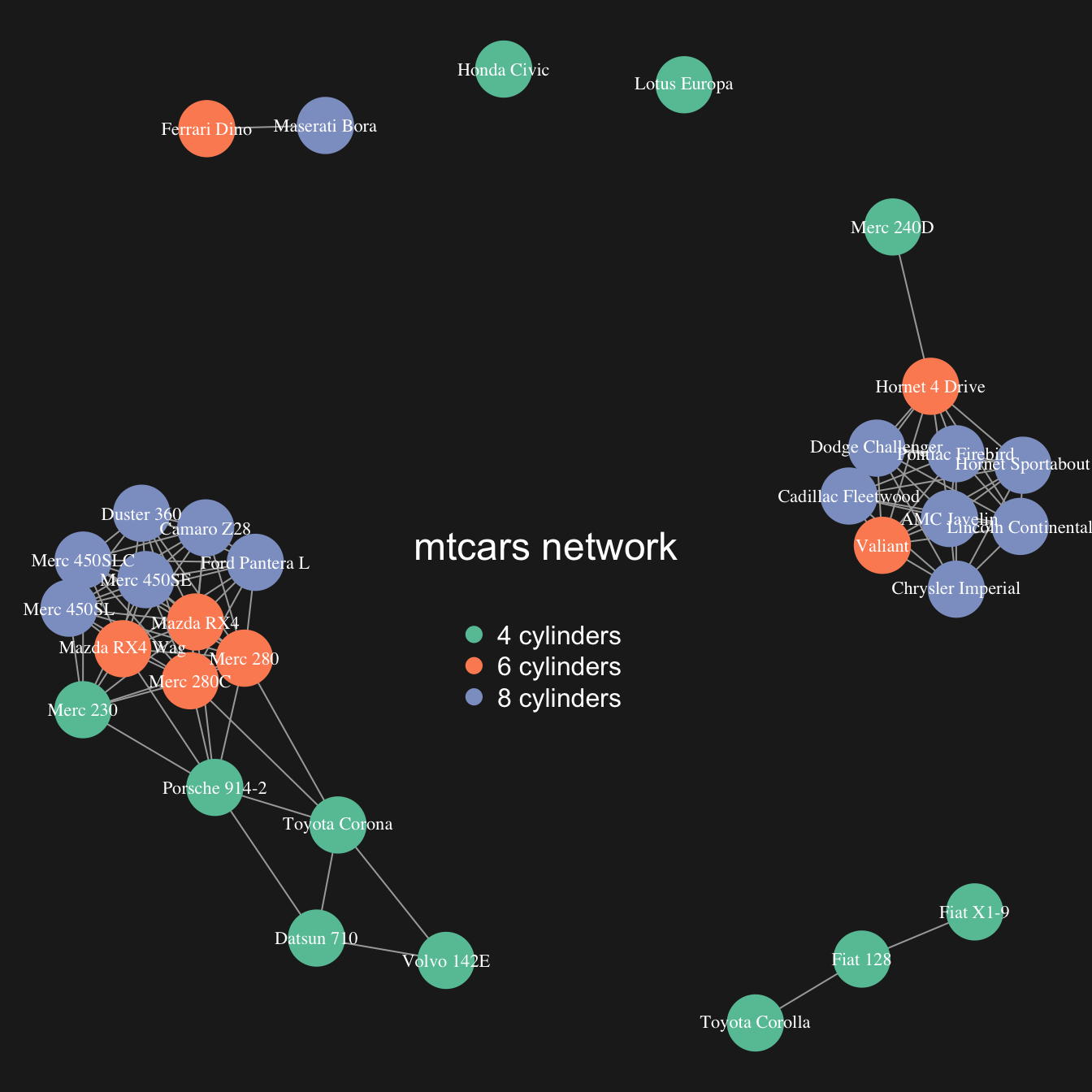# Clustering result visualization with network diagram

This post explains how to compute a correlation matrix and display the result as a network chart using R and the `igraph` package.

# Compute the correlation matrix

Consider a dataset composed by entities (usually in rows) and features (usually in columns).

It is possible to compute a correlation matrix from it. It is a square matrix showing the relationship between each pair of entity. It can be computed using correlation (`cor()`) or euclidean distance (`dist()`).

Let’s apply it to the `mtcars` dataset that is natively provided by R.

``````# library
library(igraph)

# data

# Make a correlation matrix:
mat <- cor(t(mtcars[,c(1,3:6)]))``````

# Basic network diagram

A correlation matrix can be visualized as a network diagram. Each entity of the dataset will be a node. And 2 nodes will be connected if their correlation or distance reach a threshold (`0.995` here).

To make a `graph` object from the correlation matrix, use the `graph_from_adjacency_matrix()` function of the `igraph` package. If you’re not familiar with `igraph`, the network section is full of examples to get you started.``````# Keep only high correlations
mat[mat<0.995] <- 0

# Make an Igraph object from this matrix:
network <- graph_from_adjacency_matrix( mat, weighted=T, mode="undirected", diag=F)

# Basic chart
plot(network)``````

# Customization

The hardest part of the job has been done. The chart just requires a bit of polishing for a better output:

• customize node, link, label and background features as you like
• map the node feature to a variable (`cyl` here). It gives an additional layer of information, allowing to compare the network structure with a potential expected organization.``````# color palette
library(RColorBrewer)
coul <- brewer.pal(nlevels(as.factor(mtcars\$cyl)), "Set2")

# Map the color to cylinders
my_color <- coul[as.numeric(as.factor(mtcars\$cyl))]

# plot
par(bg="grey13", mar=c(0,0,0,0))
set.seed(4)
plot(network,
vertex.size=12,
vertex.color=my_color,
vertex.label.cex=0.7,
vertex.label.color="white",
vertex.frame.color="transparent"
)

# title and legend
text(0,0,"mtcars network",col="white", cex=1.5)
legend(x=-0.2, y=-0.12,
legend=paste( levels(as.factor(mtcars\$cyl)), " cylinders", sep=""),
col = coul ,
bty = "n", pch=20 , pt.cex = 2, cex = 1,
text.col="white" , horiz = F)``````

Last but not least, control edges with arguments starting with `edge.`.``````plot(network,
edge.color=rep(c("red","pink"),5),           # Edge color
edge.width=seq(1,10),                        # Edge width, defaults to 1
edge.arrow.size=1,                           # Arrow size, defaults to 1
edge.arrow.width=1,                          # Arrow width, defaults to 1
edge.lty=c("solid")                           # Line type, could be 0 or “blank”, 1 or “solid”, 2 or “dashed”, 3 or “dotted”, 4 or “dotdash”, 5 or “longdash”, 6 or “twodash”
#edge.curved=c(rep(0,5), rep(1,5))            # Edge curvature, range 0-1 (FALSE sets it to 0, TRUE to 0.5)
)``````

# All customization

Of course, you can use all the options described above all together on the same chart, for a high level of customization.``````par(bg="black")

plot(network,

# === vertex
vertex.color = rgb(0.8,0.4,0.3,0.8),          # Node color
vertex.frame.color = "white",                 # Node border color
vertex.shape="circle",                        # One of “none”, “circle”, “square”, “csquare”, “rectangle” “crectangle”, “vrectangle”, “pie”, “raster”, or “sphere”
vertex.size=14,                               # Size of the node (default is 15)
vertex.size2=NA,                              # The second size of the node (e.g. for a rectangle)

# === vertex label
vertex.label=LETTERS[1:10],                   # Character vector used to label the nodes
vertex.label.color="white",
vertex.label.family="Times",                  # Font family of the label (e.g.“Times”, “Helvetica”)
vertex.label.font=2,                          # Font: 1 plain, 2 bold, 3, italic, 4 bold italic, 5 symbol
vertex.label.cex=1,                           # Font size (multiplication factor, device-dependent)
vertex.label.dist=0,                          # Distance between the label and the vertex
vertex.label.degree=0 ,                       # The position of the label in relation to the vertex (use pi)

# === Edge
edge.color="white",                           # Edge color
edge.width=4,                                 # Edge width, defaults to 1
edge.arrow.size=1,                            # Arrow size, defaults to 1
edge.arrow.width=1,                           # Arrow width, defaults to 1
edge.lty="solid",                             # Line type, could be 0 or “blank”, 1 or “solid”, 2 or “dashed”, 3 or “dotted”, 4 or “dotdash”, 5 or “longdash”, 6 or “twodash”
edge.curved=0.3    ,                          # Edge curvature, range 0-1 (FALSE sets it to 0, TRUE to 0.5)
)``````

Related chart types

## Contact

This document is a work by Yan Holtz. Any feedback is highly encouraged. You can fill an issue on Github, drop me a message on Twitter, or send an email pasting yan.holtz.data with gmail.com.